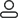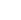LanguageLogin|Sign up
Blog >> Blog Details Page
Principle of formation of PCB board wire impedance interference
Posted:05:36 PM December 18, 2018 writer: G

A certain wire on a printed circuit board can be regarded as a regular rectangular copper strip. We take a length of 10cm, a width of 1.5mm, and a thickness of 50μm as an example. The impedance can be seen by calculation. .

The wire resistance can be calculated by the formula:

R=ρL/s (Ω)

026Ω。 The L is the wire length (m), s is the wire cross-sectional area (square mm), ρ is the resistivity ρ = 0.2. By calculation, the wire resistance value is about 0. 026 Ω.

The inductance of the wire is 0. 08μH, and the wire has a self-inductance of 0. 8μH / m, then the wire of 10cm long has an inductance of 0. 08μH. Then we can find the inductive reactance exhibited by the copying wire by the following formula:

XL=2πfL

Where π is a constant, f is the frequency (Hz) of the wire passing signal, and L is the self-inductance (H) of the wire per unit length. In this way we can calculate the inductive reactance of the wire at low and high frequencies:

When f=10MHz, XL=6. 28×10×103×0. 08×10-6≈0. 005Ω

When f=30MHz, XL=6. 28×30×106×0. 08×10-6≈16Ω

From the above formula, we can see that the wire resistance is higher than the wire inductance in the low frequency signal transmission, and the wire inductance is much larger than the wire resistance in the high frequency signal.

633 1 0 1
• PCB
Prototype
• PCB
Assembly
• SMD
Stencil
 Dimensions: (mm) × Quantity: (pcs) 5 5 10 15 20 25 30 40 50 75 100 120 150 200 250 300 350 400 450 500 600 700 800 900 1000 1500 2000 2500 3000 3500 4000 4500 5000 5500 6000 6500 7000 7500 8000 9000 10000 Other Quantities:(quantity*length*width is greater than 10㎡) OK Layers: 1 2 4 6 8 10 12 Thickness: 0.6 mm 0.8 mm 1.0 mm 1.2 mm 1.6 mm 2.0 mm 2.5 mmQuote now